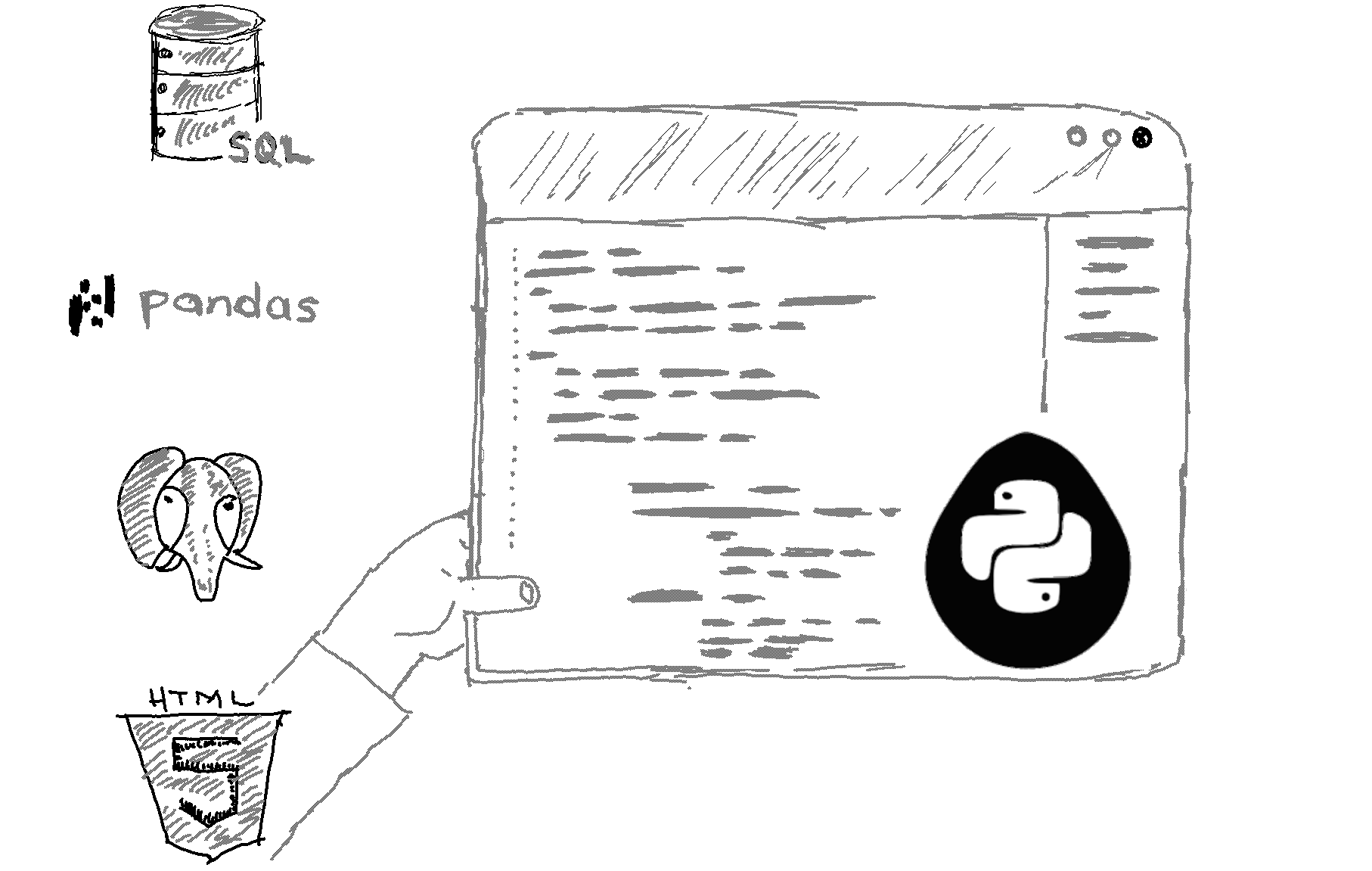# Here is how to determine the type of an object in Python.

```x = "hello"
y = [1, 2, 3]
z = (1, 2, 3)
a = {1, 2, 3}
b = 1

print(type(x))  # Output: <class 'str'>
print(type(y))  # Output: <class 'list'>
print(type(z))  # Output: <class 'tuple'>
print(type(a))  # Output: <class 'set'>
print(type(b))  # Output: <class 'int'> ```

Output
```<class 'str'>
<class 'list'>
<class 'tuple'>
<class 'set'>
<class 'int'>```

Explanation

To determine the type of an object in Python, you can use the type function. The type function returns the type of the object as a class object. The output of type is a string that specifies the type of the object. In the example above, x is a string, y is a list, z is a tuple, a is a set, and b is an integer.

You can also use the isinstance function to check if an object is an instance of a particular class or type. Here is an example of how to use isinstance to check the type of an object:

```x = "hello"
y = [1, 2, 3]

# Check if x is a string
if isinstance(x, str):
print("x is a string")
else:
print("x is not a string")

# Check if y is a list
if isinstance(y, list):
print("y is a list")
else:
print("y is not a list")```
The output of this code will be:

```x is a string
y is a list
```
Both type and isinstance are useful for checking the type of an object in Python. You can choose the one that best fits your needs.

Related HowTos
##### Recommended Course

Learn Flask development and learn to build cool apps with our premium Python course on Udemy.